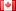# Revision history [back]

### NUMERICAL VALUE

Let's say:
z = solve(gen_legendre_P(2, 0, x) == 0, x);

$$z = [x = -1/3 * sqrt(3), x = 1/3 * sqrt(3)]$$

How can I make numerical value of that?

numerical_approx(z)
N(z)
n(z)
z.n()
RR(z)

DON'T WORK!2 No.2 RevisionDSM5192 ●21 ●68 ●111

### NUMERICAL VALUEconverting symbolic solve output to numbers [was "numerical value"]

Let's say:
z = solve(gen_legendre_P(2, 0, x) == 0, x);

$$z = [x = -1/3 * sqrt(3), x = 1/3 * sqrt(3)]$$

How can I make numerical value of that?

numerical_approx(z)
N(z)
n(z)
z.n()
RR(z)

DON'T WORK!

### converting symbolic solve output to numbers [was "numerical value"]

Let's say:
z = solve(gen_legendre_P(2, 0, x) == 0, x);
say:

$$z = [x = -1/3 * sqrt(3), x = 1/3 * sqrt(3)]$$

How can I make numerical value of that?

numerical_approx(z)
N(z)
n(z)
z.n()
RR(z)

DON'T WORK!4 retagged

### converting symbolic solve output to numbers [was "numerical value"]

Let's say:

$$z = [x = -1/3 * sqrt(3), x = 1/3 * sqrt(3)]$$

How can I make numerical value of that?

numerical_approx(z)
N(z)
n(z)
z.n()
RR(z)

DON'T WORK!